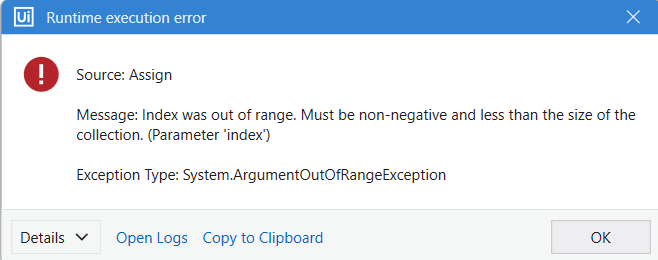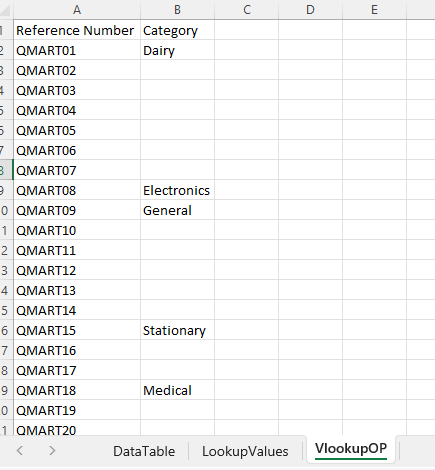# VLookup LINQ - Multiple Columns

Hello Friends,
Data.xlsx (12.4 KB)

In attached file, we have “DataTable” and “LookupValues” sheets. we have to do Vlookup for all values in “LookupValues” sheet.

DataTable - “DataTbl” variable
LookupValues - “lookup_values” variable

Below is the output table - “OP_DT”

My LINQ :-

(
From R1 In DataTbl.AsEnumerable
Let Check = lookup_values.AsEnumerable.Where(Function(R2) R2(0).ToString.Trim.Equals(R1(1).ToString.Trim)).ToList
Let Check_Flag = If(Check.Count() > 0, Check(0), Check(1))
Let Flag = {R1(1), Check_Flag}
).CopyToDataTable

However its giving below error :

May i know what i am missing here ?

Hi @Neal369 ,

I guess you are missing the below marked line in your screenshot,

`Let Check_Flag = If(Check.Count() > 0, Check(0), Check(1))` → This line is not same as in your expression editor.

You have 5 columns in OP_DT datatable and your are adding only 2 values in below line,
Let Flag = {R1(1), Check_Flag}

So please update the above line with,
Let Flag = {R1(1), Check_Flag,String.Empty,String.Empty,String.Empty}

1 Like

@Manish540

I tried both ways but i am getting error in both cases,

Let Check_Flag = If(Check.Count() > 0, Check(0), Check(1))

This line gives me below run time error,Hi @Neal369 ,

If your Check.Count > 0 then you have to use below code,
`Let Check_Flag = If(Check.Count() > 0, Check(0), "List Count is Zero")`
And you can’t use ‘Check(1)’ in Else part of If condition, if your Check.Count is zero.

1 Like

@Manish540 Its still giving error,
We need data for all the target columns in build data columns and their sequence is not same as that in source data table.

Attaching project folder,

022_VLookup using LINQ.zip (13.2 KB)

Hi @Neal369 ,

I have fixed the issue, please use this below code in your assign and let me know,

``````(
From R1 In DataTbl.AsEnumerable
Let Check = lookup_values.AsEnumerable.Where(Function(R2) R2(0).ToString.Trim.Equals(R1(1).ToString.Trim)).ToList()
Let Check_Flag = If(Check.Count() > 0, Check(0).ToString, String.Empty)
Let Flag = {R1(1).ToString,Check_Flag}
).CopyToDataTable
``````

1 Like

Hi @Neal369 ,

If you want to get “Category” column value from DataTbl table to OP_DT table for matching ‘Reference Number’ in lookup_values then use this below query,

``````(
From R1 In DataTbl.AsEnumerable
Let Check = lookup_values.AsEnumerable.Where(Function(R2) R2(0).ToString.Trim.Equals(R1(1).ToString.Trim)).ToList()
Let Check_Flag = If(Check.Count() > 0, R1("Category").ToString, String.Empty)
Let Flag = {R1(1).ToString,Check_Flag}
).CopyToDataTable
``````

Output Screenshot:Hope this might help you1 Like

Hi @Manish540
This is good and helps, but is there any approach/way to optimize the query so that it gives output only for required “Reference numbers” as mentioned “lookup_values” table, also it should give output for all target columns mentioned in “OP_DT” table in 1 go.

Hi @Neal369 ,

Do you require a Linq approach to perform this strictly, it does seem that you would want to perform a Join Operation (Inner Join), we can do this using the `Join Datatables` Activity.

If already tried with `Join Datatables` Activity, let us know what was not working with it.

Then from the resultant Datatable we can keep only the required columns.

1 Like

@supermanPunch
That’s correct, with Join Datatables activity, it becomes very easy,
But i am trying by using LINQ approach.

Maybe you could also try with the below :

``````OP_DT = (From R1 In DataTbl.AsEnumerable
Join R2 In lookup_values.AsEnumerable
On R1("Reference Number").toString.Trim Equals R2("Reference Number").ToString.Trim
Let ra = R1.ItemArray
``````

For this to be properly executed, you would need to modify a previous step, where we wouldn’t need to use `Build Datatable` Activity to prepare the columns, rather you could create an array of String which contains the column names that you would want to preserve in the output datatable. We can name the array of string variables as `requiredColumns`, so you would need to do the below using an `Assign` Activity.

``````requiredColumns = {"Reference Number","Category","Sale Price","Sales Person","Region"}
``````

So Instead of the `Build Datatable` activity, you could use the below expression in the `Assign` Activity. Make sure to do the below after the `DataTbl` is read using `Read Range` activity.

``````OP_DT = DataTbl.Clone
``````

At the last, after the Linq Expression is used, you can use the below Expression to preserve only the required columns in the Output Datatable.

``````OP_DT = OP_DT.DefaultView.ToTable(false,requiredColumns)
``````

Maybe this is a re-work that you would need to do, but this is also an alternate way.

Also, as I have taken a look at the data that you have, it seems in the Look up values sheet, there is only one column, this would also mean that we could straight up perform a Filtration on the DataTable sheet and get the required output.